# 洋金花生物碱提取研究Study on the Extraction of Alkaloids from Flos Daturae

DOI: 10.12677/PI.2019.83014, PDF, HTML, XML, 下载: 422  浏览: 1,579  国家自然科学基金支持

Abstract: Objective: To study the effects of two extraction methods on the extraction rate of total alkaloids from Flos Daturae, and to compare the distribution of alkaloids in the peel and grain of Flos Daturae and to isolate and purify the total alkaloids from Flos Daturae. Methods: The concentration of alkaloids in grains and shells was compared by thin layer chromatography (TLC). The alkaloids in the Flos Daturae were separated and purified by silica gel column chromatography. Results: The con-tent of alkaloids in the seeds of Flos Daturae was higher than that in the shell, and the content of alkaloids in the shell was very low (<0.5%). The total alkaloid extraction rate in the seeds of Flos Daturae was 3.3%, microwave. The extraction method was 1.4%. Conclusion: The alkaloids in the Flos Daturae are mainly distributed in the grain; the alkaloids in the Flos Daturae seeds are extracted by reflux method, the operation is simple, the solvent is used less, the conditions are mild, and the total alkaloid extraction rate is high. Considering all aspects, it is more appropriate to extract the Agrobacterium alkaloids by reflux method.

1. 引言

2. 材料及仪器

2.1. 材料

2017年10月在贵州大学校园内采摘洋金花果实，并将其晾干。在使用前将籽粒与壳分开，并将壳剪碎成3~5 cm大小。

2.2. 试剂

80%乙醇溶液、蒸馏水、100~200目硅胶粉、200~300目的硅胶粉

2.3. 仪器

3. 生物碱的提取

3.1. 洋金花籽生物碱水微波提取

①称取洋金花籽10.0 g + 150 mL → 微波加热1小时

(加热方式：将10.0 g洋金花籽与水放在大烧杯内，放入微波炉中中火加热至沸腾，拿出冷却，再放入微波炉内中火加热沸腾，冷却，反复持续1小时)

② 选一大小适宜的干燥的蒸发皿，在电子天平中准确称取其质量。将大烧杯内的提取液倒入蒸发皿内，放在水浴锅中蒸干至药膏，再称取粗提物与蒸发皿的质量，结果如下。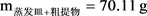${\text{m}}_{蒸发皿}=70.11\text{\hspace{0.17em}}\text{g}$

${\text{m}}_{粗提物}=0.\text{14}\text{\hspace{0.17em}}\text{g}$

3.2. 洋金花籽生物碱回流提取

① 称取洋金花籽10.0 g + 150 mL 80%乙醇 → 回流提取1小时

② 选一大小适宜的干燥的坩埚，在电子天平中准确称取其质量。将圆底烧瓶内的提取液倒入坩埚内，放在水浴锅中蒸干至药膏，再称取粗提物与坩埚的质量，结果如下，提取率比较结果见表1

${\text{m}}_{蒸发皿+粗提物}=\text{66}.\text{17}\text{\hspace{0.17em}}\text{g}$

${\text{m}}_{蒸发皿}=\text{66}.\text{5}0\text{\hspace{0.17em}}\text{g}$

${\text{m}}_{蒸发皿}=0.\text{33}\text{\hspace{0.17em}}\text{g}$Table 1. Comparison of alkaloid extraction rates of Flos Lonicerae

3.3. 洋金花壳水提取

① 称取洋金花壳10.0 g + 400 mL → 微波中火加热1小时，(加热方式：将10.0 g洋金花壳与300 mL水放在大烧杯内，放入微波炉中中火加热至沸腾，拿出冷却，再放入微波炉中火内加热沸腾，加热到最后大烧杯内水几乎蒸干，再加入100 mL水，加热沸腾，冷却，反复持续1小时)。同上操作，结果如下。

${\text{m}}_{蒸发皿+粗提物}=\text{53}.\text{14}\text{\hspace{0.17em}}\text{g}$

${\text{m}}_{蒸发皿}=\text{54}.0\text{4}\text{\hspace{0.17em}}\text{g}$

${\text{m}}_{粗提物}=0.\text{9}0\text{\hspace{0.17em}}\text{g}$

3.4. 洋金花壳醇提取

① 称取洋金花壳10.0 g + 300 mL 80%乙醇 → 回流提取1小时，同上操作，结果如下，提取率比较结果见表2

${\text{m}}_{蒸发皿+粗提物}=\text{54}.0\text{4}\text{\hspace{0.17em}}\text{g}$

${\text{m}}_{坩埚}=\text{53}.\text{14}\text{\hspace{0.17em}}\text{g}$

${\text{m}}_{蒸发皿}=0.\text{79}\text{\hspace{0.17em}}\text{g}$Table 2. Comparison of extraction rates of alkaloids from the golden flower shell

4. 洋金花粗提物生物碱的定性分析

4.1. 洋金花籽粒粗提物TCL鉴定(注：从左到右分别为洋金花籽生物碱醇粗提物、洋金花籽生物碱水粗提物)

Figure 1. Dendrocalamopsis alkaloids analysis thin layer chromatography

4.2. 洋金花壳粗提取物TCL鉴定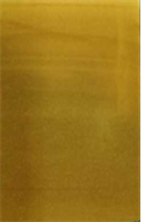(注：从左到右分别为洋金花壳生物碱醇提取物、洋金花生物碱壳水提取物)

Figure 2. Thin layer chromatography of alkaloids analysis

5. 洋金花生物碱柱层析极性研究

5.1. 装柱

5.2. 洗脱

②二氯甲烷:甲醇 = 180:20 mL

③二氯甲烷:甲醇 = 150:50 mL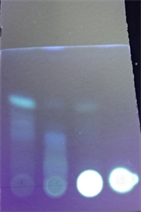Figure 3. Sample analysis thin layer chromatography (254 nm)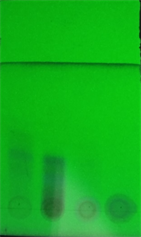Figure 4. Sample analysis thin layer chromatography (365 nm)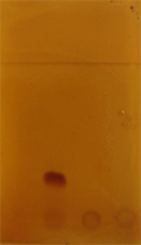(注：从左往右分别为使用洗脱液①、②、原始样液、③的洗脱产物)

Figure 5. Sample analysis thin layer chromatography

6. 结论

  尚天翠. 超声波法提取曼陀罗种子总生物碱的工艺优化[J]. 辽宁化工, 2016, 45(5): 537-539.  牛丽颖, 王继业, 遭秀莲, 等. 薄层色谱法测定洋金花中东莨菪碱的含量[J]. 河北中医学报, 2004, 19(3): 25.  杨炳友, 周永强, 刘艳, 等. 洋金花中生物碱类成分的分离与鉴定[J]. 中医药信息, 2017, 34(3): 5-8.  李万林. 洋金花种子中有效成分提取工艺的研究[J]. 广东饲料, 23(3): 24-26.  彭拓华, 钟世顺, 张少俊, 等. 三种提取方法提取洋金花生物碱的比较研究[J]. 中成药, 2012, 34(5): 832-835.  朱月琴, 张弦, 殷放宙. HPLC法测定洋金花及其总生物碱中东莨菪碱的含量[J]. 海峡药学, 2006, 18(1): 95-97.  李振宇, 杨炳友, 舒尊鹏. 洋金花化学拆分组分的性味药理学评价[J]. 中医药信息, 2011, 28(6): 8-13.